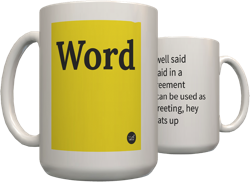Top definition
An example that illustrates a solution but is not actually accuate or proof of the theory.
"2 x 2 = 4 and 2 + 2 = 4, therefore multiplication is the same thing as addition."

Even though 2 x2 DOES equal 4, it is a solution that just happens to fit but is not proof that multiplication is the same thing as addition, since multiplying two values usually results in a figure much different than adding those two values (such as 2 x 20 = 40, but 2 + 20 = 22).

Usage: "No sorry, that doesn't prove anything. That's just a Mickey Mouse problem."
by Venus Libertina November 10, 2010Get a Mickey Mouse problem mug for your cousin Vivek.